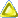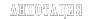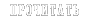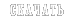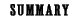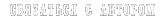new! - Download the book in english (pdf, 672 kb) or visit Los Alamos library to download it in PostScript or other formats.

#### Eugene Perchik. Methodology of syntheses of knowledge: overcoming incorrectness of the problems of mathematical modelingJ. Hadamard's ideas about the correct statement of the problems of mathematical physics have been analyzed, and also various interpretations of the directly related Banach theorem about the inverse operator has been followed in this context. The contemporary apparatus of mathematical modeling is shown to be in a drastic contradiction with concepts of J. Hadamard, S. Banach and a number of other outstanding scientists in the sense that the priority is given to the realization of inefficient algorithms, which actually means that incorrectly stated problems are believed to be adequate to real phenomena.

A new method is developed for solving problems traditionally associated with the first-order Fredholm integral equations. It is essentially based on the constructive use of the possibilities to ensure the conditions of correctness inherent in functional space l2. The smoothing of information because of integration is taken into account by a special composition, which explicitly includes a sought-for function and is infinitely small in the space L2. The finite error because of the fact that data are obtained by measurements may also be represented in the same manner. By relatively simple transformation the mentioned circle of problems is reduced to the solution of second-order Fredholm integral equations with properties most favorable for the numerical realization.

The possibility is shown to extend the approach suggested for a wide circle of problems that may be reduced to the first-order Fredholm equations; these are linear boundary-value and initial-boundary-value problems with variable coefficients, non-canonical domain of definition and other peculiarities complicating their solution. A basically similar apparatus have been developed for solving initially-boundary-value problems for nonlinear differential equations of evolutionary type. Also considered are boundary-value problems for an essentially nonlinear equation and an equation of mixed type, for various cases of nonlinearities in a boundary condition and small factors at the highest derivative, for the inverse problem of recovery of a variable coefficient of differential operator and a problem of Stephan type.

Conclusions about cause-and-effect relations are argued to be essentially illegitimate when the solution of a problem is traced in the long run to a primitive renaming of known and unknown functions of a corresponding direct problem. The approach suggested may be considered as a constractive realization of J. Hadamard's opinions that physically meaningful problems always have correct statements.